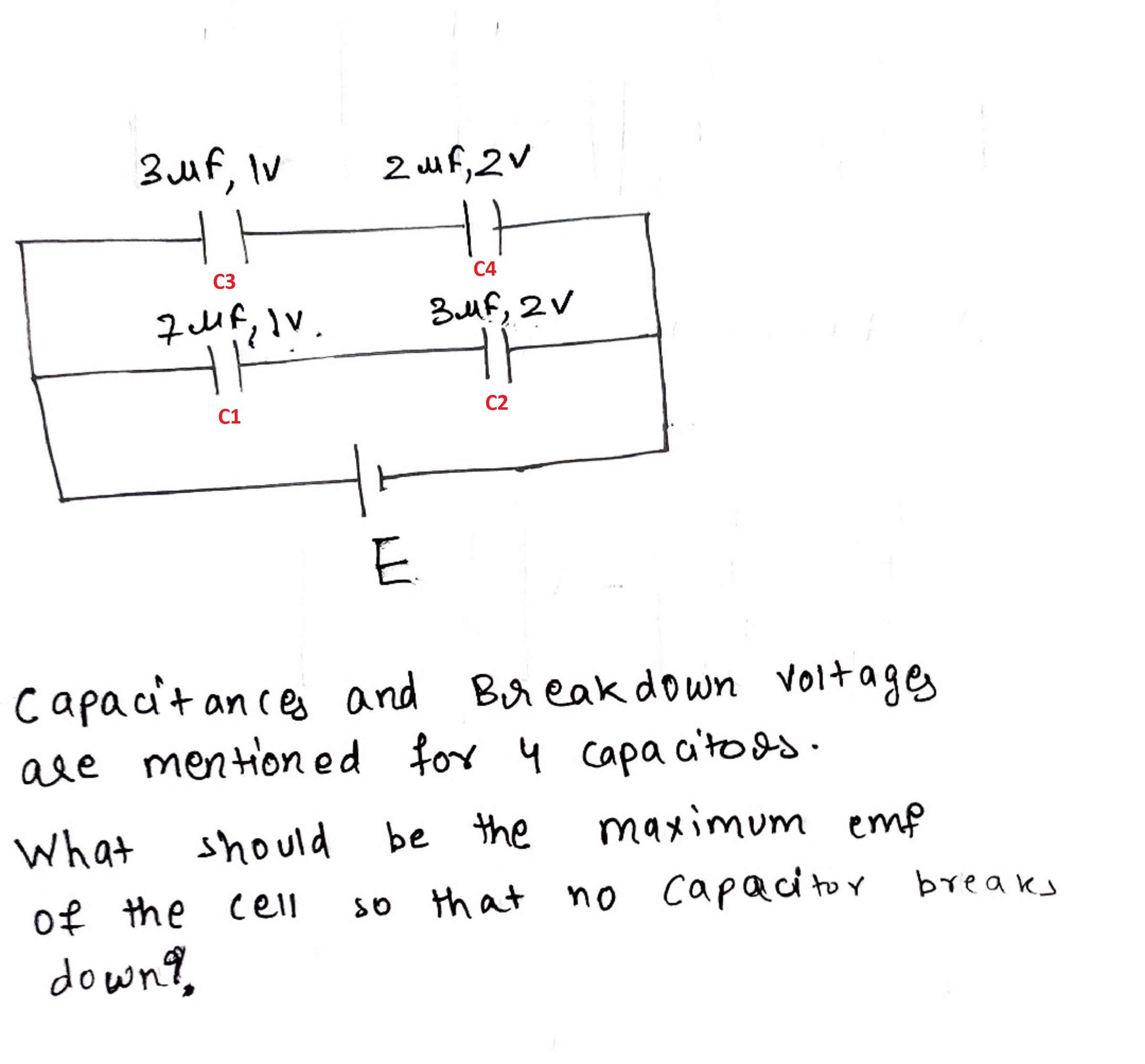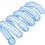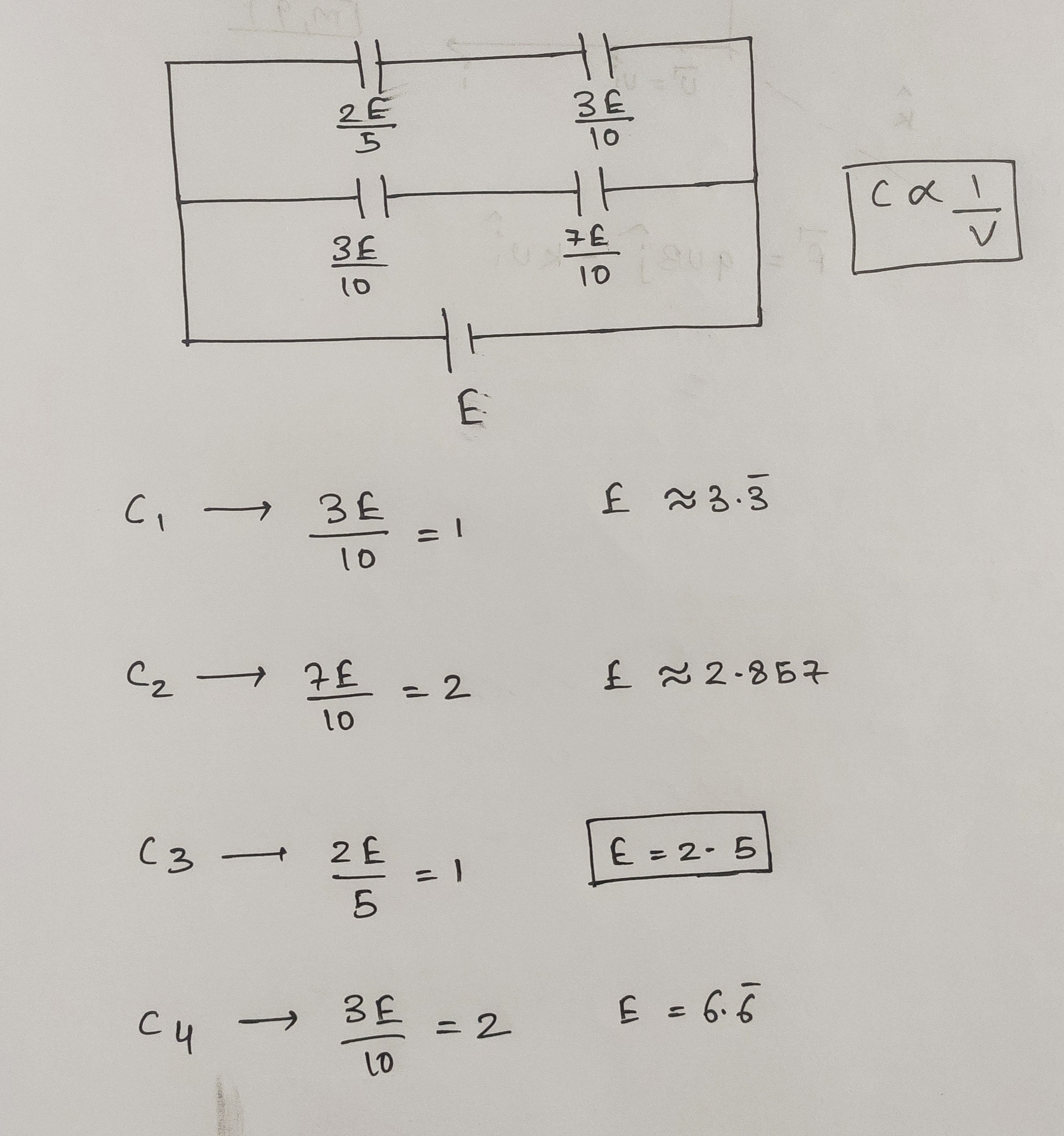# Capacitor Over-voltage Exercise

Here is a problem I received recentlyThe first basic principle is the relationship between the capacitor charge and voltage:

$Q = C V \\ V = \frac{Q}{C}$

The second basic principle is that since capacitors 1 and 2 are in series, they have the same charge $Q_{12}$. Capacitors 3 and 4 also have the same charge $Q_{34}$.

These two principles yield the following:

$E = \frac{Q_{12}}{C_1} + \frac{Q_{12}}{C_2} \\ E = \frac{Q_{34}}{C_3} + \frac{Q_{34}}{C_4}$

The procedure is therefore:

1) Sweep $E$ over a range
2) For each value of $E$, solve for $Q_{12}$ and $Q_{34}$
3) Then for each $E$, solve for the capacitor voltages after the charges are known
4) Stop sweeping $E$ when any of the capacitor voltages exceeds its limit

As it turns out, $E = 2.5$ is the source voltage at which the first capacitor fails.

  1 2 3 4 5 6 7 8 9 10 11 12 13 14 15 16 17 18 19 20 21 22 23 24 25 26 27 28 29 30 31 32 33 34 35 36 37 38 39 40 41 42 43 44 45 46 47 48 49 50 51 import math u = 10.0**(-6.0) C1 = 7.0*u C2 = 3.0*u C3 = 3.0*u C4 = 2.0*u V1max = 1.0 V2max = 2.0 V3max = 1.0 V4max = 2.0 dE = 10.0**(-5.0) ############################## E = 0.0 flag = 0 while flag == 0: Q12 = E/(1.0/C1 + 1.0/C2) Q34 = E/(1.0/C3 + 1.0/C4) V1 = Q12/C1 V2 = Q12/C2 V3 = Q34/C3 V4 = Q34/C4 if V1 > V1max: flag = 1 if V2 > V2max: flag = 1 if V3 > V3max: flag = 1 if V4 > V4max: flag = 1 E = E + dE ############################## print E #>>> #2.50001000001Note by Steven Chase
4 months, 1 week ago

This discussion board is a place to discuss our Daily Challenges and the math and science related to those challenges. Explanations are more than just a solution — they should explain the steps and thinking strategies that you used to obtain the solution. Comments should further the discussion of math and science.

When posting on Brilliant:

• Use the emojis to react to an explanation, whether you're congratulating a job well done , or just really confused .
• Ask specific questions about the challenge or the steps in somebody's explanation. Well-posed questions can add a lot to the discussion, but posting "I don't understand!" doesn't help anyone.
• Try to contribute something new to the discussion, whether it is an extension, generalization or other idea related to the challenge.

MarkdownAppears as
*italics* or _italics_ italics
**bold** or __bold__ bold
- bulleted- list
• bulleted
• list
1. numbered2. list
1. numbered
2. list
Note: you must add a full line of space before and after lists for them to show up correctly
paragraph 1paragraph 2

paragraph 1

paragraph 2

[example link](https://brilliant.org)example link
> This is a quote
This is a quote
    # I indented these lines
# 4 spaces, and now they show
# up as a code block.

print "hello world"
# I indented these lines
# 4 spaces, and now they show
# up as a code block.

print "hello world"
MathAppears as
Remember to wrap math in $$ ... $$ or $ ... $ to ensure proper formatting.
2 \times 3 $2 \times 3$
2^{34} $2^{34}$
a_{i-1} $a_{i-1}$
\frac{2}{3} $\frac{2}{3}$
\sqrt{2} $\sqrt{2}$
\sum_{i=1}^3 $\sum_{i=1}^3$
\sin \theta $\sin \theta$
\boxed{123} $\boxed{123}$

Sort by:

@Lil Doug This was a good one

- 4 months, 1 week ago

@Steven Chase wait, if I applied a battery of $E=2.6$ it doesn't mean that the potential across that 1st capacitor will be 2.6 , so how??
There is one more capacitor in series with first one???

- 4 months, 1 week ago

No single capacitor will get the full battery voltage

- 4 months, 1 week ago

@Steven Chase how can you say so confidently?

- 4 months, 1 week ago

It is explained in the note. Both series capacitors in each branch have the same charge. It is therefore impossible for one to have voltage without the other one also having some. So neither one can have the full source voltage across it

- 4 months, 1 week ago

@Steven Chase But how? Confusion is killing me.

- 4 months, 1 week ago

@Steven Chase voltage will get divided in both capacitor in each branch? So it will be less than 2.6.isn't it?

- 4 months, 1 week ago

Yes, it is divided across both, but not evenly

- 4 months, 1 week ago

@Steven Chase it will be like $(2,0.6) , (1.9, 0.7), (1,1.6)$ so what?

- 4 months, 1 week ago

So sweep the source voltage upward until one of the capacitors reaches its limiting voltage

- 4 months, 1 week ago

@Steven Chase so, can you show by sweeping?

- 4 months, 1 week ago

It's in the note

- 4 months, 1 week ago

@Steven Chase why it getting so much hard.
Now I will show my work, let the charge in that $7 uF$ be x
Then, $E=\frac{x}{7}+\frac{x}{3}$
Which gives $x=5.46$ Can you resolve it, where I am lacking.?

- 4 months, 1 week ago

Once you have calculated the charge, you can calculate the capacitor voltages

- 4 months, 1 week ago

@Steven Chase This is the ideal explanation, I will give if anyone will ask me this problem- 4 months, 1 week ago

@Steven Chase and the 3rd capacitor fails, not the first one

- 4 months, 1 week ago

@Steven Chase Try my new problem.. You have also love affair with this types problem, therefore I posted.

- 4 months, 1 week ago

@Steven Chase have a look on 37th problem. Please

- 4 months, 1 week ago

@Steven Chase seems that you are online and free. I have some doubts can you help me ? Please

- 3 months, 1 week ago

@Steven Chase by the way what is the salary of a person in NASA?

- 3 months, 1 week ago